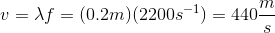# MCAT Physical : Velocity and Waves

## Example Questions

### Example Question #174 : Mcat Physical Sciences

Sound traveling at a velocity, V1, through a certain medium will travel at what velocity through a medium of twice the density?

½ V1

¼ V1

2 V1

It depends on the medium’s resistance to compression

It depends on the medium’s resistance to compression

Explanation:

The speed of sound depends on both the medium’s density and resistance to compression. We do not have enough information to solve for V2 in terms of V1.

### Example Question #175 : Mcat Physical Sciences

At a local concert, a speaker is set up to produce low-pitched base sounds with a frequency range of 20Hz to 200Hz, which can be modeled as  sine waves. In a simplified model, the sound waves the speaker produces can be modeled as a cylindrical pipe with one end closed that travel through the air at a velocity of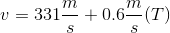, where T is the temperature in °C.

How does the speed of sound in the summer (30­oC) compare to the speed of sound in the winter (9oC)?

Speed of sound in summer is equal to the speed of sound in winter

Speed of sound in summer is 1.04 times the speed of sound in winter

Speed of sound in summer is 1.2 times the speed of sound in winter

Speed of sound in summer is 0.81 times the speed of sound in winter

Speed of sound in summer is 1.04 times the speed of sound in winter

Explanation:

This question asks us to use information provided in the paragraph about how the speed of sound varies with temperature. We can see from the relationship provided that in warmer temperatures the speed of sound is faster. This intuitively makes sense—hotter temperatures mean that air molecules are moving around more, and thus have less resistance to compression or rarefaction by a propagating sound wave. Now that we have a qualitative understanding, we need to compute the ratio of the velocities.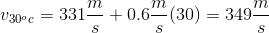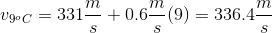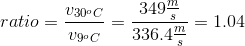### Example Question #1 : Velocity And Waves

At a local concert, a speaker is set up to produce low-pitched base sounds with a frequency range of 20Hz to 200Hz, which can be modeled as  sine waves. In a simplified model, the sound waves the speaker produces can be modeled as a cylindrical pipe with one end closed that travel through the air at a velocity of, where T is the temperature in °C.

How long would it take for a 30Hz beat to reach an audience member 100m away when the ambient temperature is 21ºC?

0.29s

0.31s

0.91s

0.11s

0.29s

Explanation:

The question asks us to determine how long it will take for a wave beat to reach an audience member at 100m away; thus, we need to calculate the velocity of the wave to determine the time.

We know from kinematics that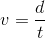. This can be rearranged to solve for t: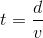.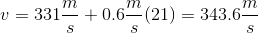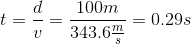### Example Question #171 : Ap Physics 2

A wave produced on a string travels with a velocity of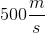. If the tension on the string is increased by a factor of four, at what speed does the wave travel?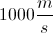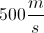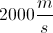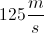Explanation:

The velocity of a wave can be obtained with the formula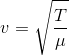, where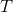is the tension in the string and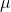is the mass per unit length of the string. Since the tension is quadrupled, the velocity will be doubled.

Let's assume that a string with tensionand a mass per unit lengthproduces a wave with velocity.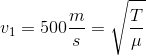If we increase the tension by a factor of four, we will get the below expression.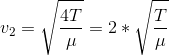We can see that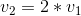, and we know that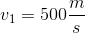.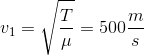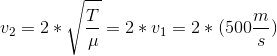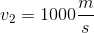### Example Question #31 : Ap Physics 2

Through which of the following would you expect a photon to travel fastest?

Vacuum

Glass

Air

Water

Vacuum

Explanation:

A photon will travel fastest through a vacuum. Photons are generally massless and can be thought of as a light wave, which travels fastest in a vacuum and slowest through a metal or solid. This can be visualized using the concept of the index of refraction, which describes the speed of light through air compared to the speed through other mediums. A vacuum will be the least dense and cause the least hindrance to a photon as it travels, thus giving it the lowest index of refraction and allowing the fastest speed of light.

### Example Question #2 : Velocity And Waves

You are out snorkling off the coast of an exotic island when a pod of whales comes swimming by. The pod is 100m away. If they emit sounds underwater with an average frequency of 2200Hz and there are 500 complete sound waves between you and the pod, how fast is the speed of sound in the water?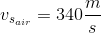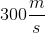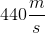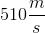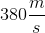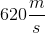Explanation:

If there are 500 waves over a distance of 100 meters, we can say that the wavelength is: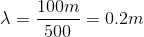Now we can use the formula for the speed of waves: